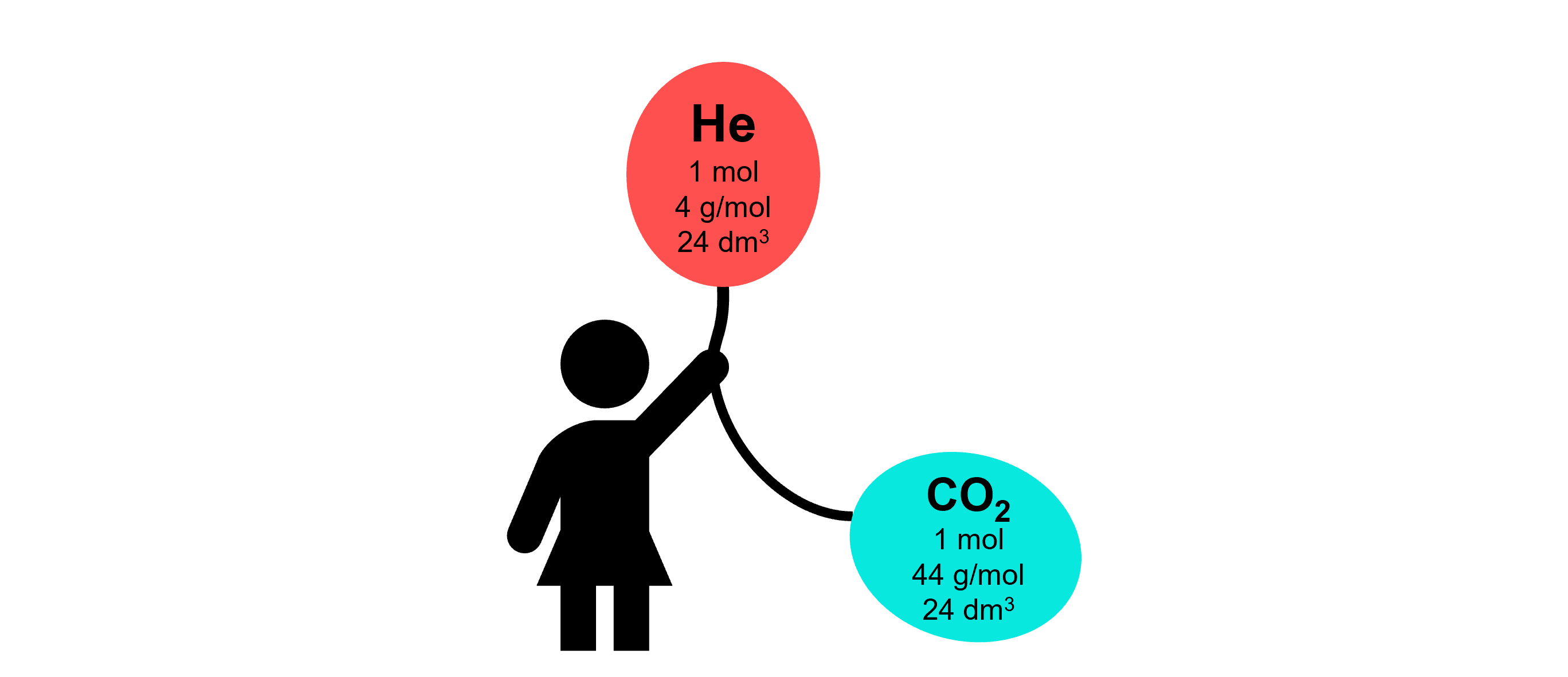## 1. Counting in moles

One mole is defined as 6×1023 particles. This is the Avogadro number, an unchanging constant. Whether you are counting hydrogen atoms, carbon dioxide molecules, or carbonate ions, a mole is always 6×1023. But as different particles have different mass, their molar mass varies.

Now let’s turn to another physical property: the volume of gases.

We know that hot air expands, occupying more space. We know that compressed gas takes up less space at high pressure. But what about the volume of different gases? Does the chemical composition of gas affect the volume it takes?

Fortunately, before we wrecked our brains thinking about this, Amedeo Avogadro racked his brain a few years before Raffles came to Singapore. He theorised that equal volumes of gases at the same pressure and temperature contain equal number of molecules, regardless of the identity of gas. So how can we test this?

## 3. The same molar volume, regardless of…Balloons with 1 mol of gas are equally inflated, with the same molar volume but different molar mass.

We can verify Avogadro’s theory by filling up a balloon with 1 mol of helium gas and another with 1 mol of carbon dioxide. Given that carbon dioxide has a heavier molar mass than helium, packing 1 mol of carbon dioxide into the same volume means that the gas is denser. Indeed, the carbon dioxide balloon sinks while helium floats.

Therefore, under similar conditions, 1 mol of different gases have the same volume. We call this the molar volume.

Molar volume is the volume of 1 mol of gas. At the room temperature of 25 °C (298 K) and atmospheric pressure of 1 atm (101000 Pa), the molar volume of any gas is 24 dm3.

## 4. Formula to convert between volume of gas and its number of moles

Just like how we can use molar mass to convert between the mass of a sample and its number of moles, we can use molar volume to convert between the volume of a gas and its number of moles.

number of moles = volume of gas in dm3 ÷ 24 (molar volume)

This formula is slightly tricky, with volume appearing multiple times. Molar volume is a constant at room temperature and pressure (rtp). It is always 24 dm3. However, the volume of gas depends on what sample you are measuring. In the exams, it will be given by the question.

## 5. Using molar volume in the lab to calculate the number of moles of a gas

Now you can convert the measurement you make in the lab to moles. Let’s say you mix magnesium strips with sulfuric acid to produce 15 mℓ of hydrogen gas. To calculate the number of moles:

• ### STEP 1: Check if the conditions are at room temperature and pressure (rtp)

Indeed, the aircon is turned on to kill the tropical heat, maintaining the temperature at 25 °C. And since the classroom isn’t atop Mount Everest, the pressure should be 1 atm.

• ### STEP 2: Convert volumes to dm3

15 mℓ = 15 cm3
15 cm3 = 15 ÷ 1000 dm3 = 0.015 dm3

• ### STEP 3: Divide by molar volume to get number of moles

No. of moles of H2 = 0.015 ÷ 24 = 6.25 × 10-4 mol